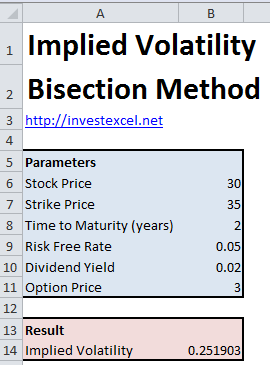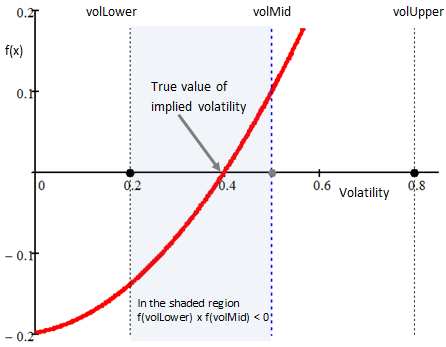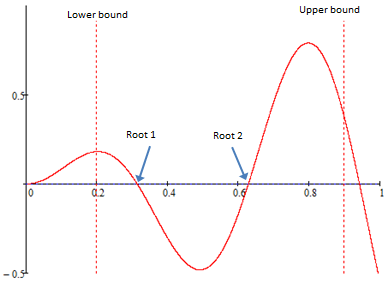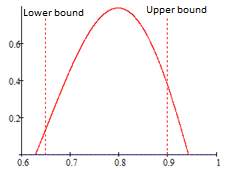# Calculate Implied Volatility with the Bisection Method

Get an Excel spreadsheet & VBA to calculate implied volatility with the bisection method. Discover how numerical bisection works, its advantages and disadvantages

The implied volatility of an option is the volatility that matches its current market price (given the governing stock price, strike price, time to maturity, risk-free rate and dividend yield). Analysts compare implied and historical volatility to judge whether the option is fairly priced.

Quantitative analysts use several numerical methods to calculate implied volatility, including Newton-Raphson iteration and numerical bisection. The latter is easy to implement, and, unlike the Newton-Raphson approach, does not need numerical derivatives in its calculation.Let’s explore the bisection algorithm in greater detail.

## Step-by-Step Guide to Numerical Bisection

Step 1
If you want to calculate the implied volatility of an option, conceptually we want to find the root of this equation

f(v) = BlackScholesCall(S, X, T, r, d, v) – Price

i.e. the value of v for which f(v) = 0.

Start by picking upper and lower bounds of the volatility (volLower and volUpper) such that f(volLower) and f(volUpper) are opposite in sign. This means that the root (i.e. the implied volatility) lies between volLower and volUpper

Step 2
Calculate a volatility that lies halfway between volUpper and volLower, i.e. volMid = (volLower + volUpper)/2

Step 3
If f(volMid) = 0 (or is less than a threshold value, called epsilonABS in the VBA), then you’ve found your implied volatility. You can stop now!

Step 4
Evaluate f(volLower) and f(volMid). If these two are opposite in sign, i.e. if f(volLower) × f(volMid) < 0, then the root lies between volLower and volMid.

If, however, f(volLower) × f(volMid) > 0, then the root lies between volMid and volUpper.Step 4
If f(volLower) × f(volMid) < 0, then let volUpper = volMid, and go back to Step 2. But if f(volLower) × f(volMid) > 0, then let volLower = volMid, and go back to Step 2.

Bisection is as simple as that, and the VBA code below implements this algorithm. It’s a robust method to calculate implied volatility. But when using bisection for other applications, you could need to account for several complications in the way your function behaves between the upper and lower bounds.

For example, a function may have two or more roots between the upper and lower search bounds.Or there may be no roots between your upper and lower search boundsThe bisection method benefits from several advantages

1. The method always converges when the function changes sign – there’s no way for the method to diverge
2. The maximum error will always be the difference between the upper and lower bounds of the volatility. By increasing the number of iterations, you can control the error; each iteration halves the error
However, the method also suffers from several disadvantages.
1. Convergence is slower than with other methods (e.g. Newton-Raphson iteration)
2. The number of iterations needed to accurately calculate the root of f(x) increases if either the initial upper or lower bounds are close to the actual root.
3. The method only works if f(x) changes sign. If the root is tangent to f(x) = 0, then the method will not converge
4. The method only works if f(x) is real and continuous between the upper and lower bounds. Numerical bisection will not work for complex-valued functions, or if it hits a discontinuity

The VBA includes two functions

• BlackScholesCall() calculates the price of a call option
• ImpliedVolatility() calculates implied volatility. It uses the bisection method to calculate volatility using BlackScholesCall()
``` Function BlackScholesCall( _ ByVal S As Double, _ ByVal X As Double, _ ByVal T As Double, _ ByVal r As Double, _ ByVal d As Double, _ ByVal v As Double) As Double Dim d1 As Double Dim d2 As Double d1 = (Log(S / X) + (r - d + v ^ 2 / 2) * T) / v / Sqr(T) d2 = d1 - v * Sqr(T) BlackScholesCall = Exp(-d * T) * S * Application.NormSDist(d1) - X * Exp(-r * T) * Application.NormSDist(d2) End Function   Function ImpliedVolatility( _ ByVal S As Double, _ ByVal X As Double, _ ByVal T As Double, _ ByVal r As Double, _ ByVal d As Double, _ ByVal Price As Double) As Double   Dim epsilonABS As Double Dim epsilonSTEP As Double Dim volMid As Double Dim niter As Integer Dim volLower As Double Dim volUpper As Double   epsilonABS = 0.0000001 epsilonSTEP = 0.0000001 niter = 0 volLower = 0.001 volUpper = 1   Do While volUpper - volLower >= epsilonSTEP Or Abs(BlackScholesCall(S, X, T, r, d, volLower) - Price) >= epsilonABS And epsilonABS <= Abs(BlackScholesCall(S, X, T, r, d, volUpper) - Price) >= epsilonABS volMid = (volLower + volUpper) / 2 If Abs(BlackScholesCall(S, X, T, r, d, volMid) - Price) <= epsilonABS Then Exit Do ElseIf ((BlackScholesCall(S, X, T, r, d, volLower) - Price) * (BlackScholesCall(S, X, T, r, d, volMid) - Price) < 0) Then volUpper = volMid Else volLower = volMid End If niter = niter + 1 Loop   ImpliedVolatility = volLower   End Function```

This spreadsheet uses the VBA given above.### 4 thoughts on “Calculate Implied Volatility with the Bisection Method”

1. Hi. Your information on calculating implied volatility using the bisection method is extremely helpful. Unfortunately, I only have Excel 2003 which doesn’t use the .xlsm format so I can’t download it. Is there any way you could send me the full text of the VBA? It’s cut off on the website and only about half the code is visible.

Thank you very much.

Lee